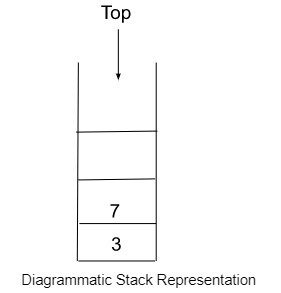# Reverse a Stack using Queue

## Introduction

Both Queue and Stack are linear data structures and are used to store data. Stack uses the LIFO principle to insert and delete its elements. A Queue uses the FIFO principle. In this tutorial, we will learn how to reverse a stack using Queue. Reversing means the last element of the Stack comes to first place and so on.

## What is Stack?

The stack in the data structure is inspired by the stack in real life. It uses LIFO (Last In First Out) logic, which means the element that enters last in the Stack will be removed first. In it, elements are inserted on the top and will be removed from the top only. Stack has only one end.

An example of a Stack in real life is a pile of newspapers. From the pile, the last placed newspaper while being removed first.

### Basic Functions of the Stack

• push() − It will insert a stack element from the top.

Syntax − stack_name.push(element type)

• pop() − It will remove elements from the top of the stack.

Syntax − stack_name.pop()

• size() − It will return the stack size.

Syntax − stack_name.size()

• top() − It returns the reference of the top element of the stack.

Syntax − stack_name.top()## What is Queue?

Queue in data structure gets its idea from the queue of real life. In this, elements are inserted at the Rear and are removed from the Front end. A queue is open to both ends and uses FIFO (First-In-First-Out) principle for its operation. This principle states that the element inserted first will be removed first from the queue.

### Basic Functions of the Queue

• push() − Insert the element on the Rear end of the Queue.

Syntax − queue_name.push(data type)

• pop() − Remove elements from the Front end of the Queue.

Syntax − queue_name.pop()

• front() −  To get the reference for the first element in the Queue.

Syntax − queue_name.front()

• size() − return size of the queue.

Syntax − queue_name.size()

## Reversing a Stack Using Queue

Let’s first understand with an example what is stack reversal.

Stack before reversing: [2,5,6,7]
Stack Reversed: [7,6,5,2]


Logic −We are taking a Stack with elements and an empty Queue. One by one pop elements from the stack and insert them into the Queue till all elements are inserted. Now, remove Queue elements and insert them into the empty Stack again. And It's done.

## Algorithm

Step 1: Insert elements into the Stack.

Step 2: Take an empty Queue.

Step 3: Push Stack elements one by one into the empty Queue.

Step 4: Now Stack is empty.

Step 5: Pop elements one by one from the queue and push into the Stack.

Step 6: Stack is reversed.

## Example

The following examples show.

#include<iostream>
#include<stack>
#include<queue>
using namespace std;
//function to reverse a queue
void reverse(queue<int> &q) {

//Declaring a stack s
stack<int> s;

//inserting Queue elements into stack
while (!q.empty()) {
s.push(q.front());
q.pop();
}

//Again pushing the elements into queue from back
while (!s.empty()) {
q.push(s.top());
s.pop();
}
}

void printQueue(queue<int> q) {

while(!q.empty()) {
cout<<q.front()<<" ";
q.pop();
}
cout<<endl;
}

int main() {
queue<int> q;
//pushing elements into the Queue
for(int i=1;i<=5;i++) {
q.push(i);
}
cout<<"Initial Queue is: ";
printQueue(q);

reverse(q);

cout<<"Reversed Queue is: ";
printQueue(q);
}


## Output

Initial Queue is: 1 2 3 4 5
Reversed Queue is: 5 4 3 2 1


## Conclusion

We can easily reverse a stack by using a Queue. We insert the stack elements into the Queue and re-inserting the Queue elements into the stack again. I hope you find this method easy to understand and implement.